## 2.1 EXPERIMENT, SAMPLE SPACE, AND EVENTS

Each probabilistic situation that we wish to analyze can be viewed in the context of an experiment. By experiment we mean any nondeterministic process that has a number of distinct possible outcomes. Thus, an experiment is first characterized by a list of possible outcomes. A particular performance of the experiment, sometimes referred to as an experimental trial, yields one and only one of the outcomes.

The finest-grained list of outcomes for an experiment is the sample space of the experiment. Examples of sample spaces are:

1. {heads, tails) in the simple toss of a coin.

2. [1, 2, 3 .... 1, describing the possible number of fire alarms in a city during a year.

3. {0x10, 0y10), describing the possible locations of required on-the-scene social services in a city 10 by 10 miles square.

As these three examples indicate, the number of elements or points in a sample space can be finite, countably infinite, or noncountably infinite. Also, the elements may be something other than numbers (e.g., "heads" or "tails").

Probabilistic analysis requires considerable manipulation in an experiment's sample space. For this, we require knowledge of the algebra of events, where an event is defined to be a collection of points in the sample space. A generic event is given an arbitrary label, such as A, B, or C. Since the entire sample space defines the universe of our concerns, it is called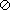, for universal event. An event containing no points in the sample space is called o, the empty event (or null set). There are three key operations in the algebra of events: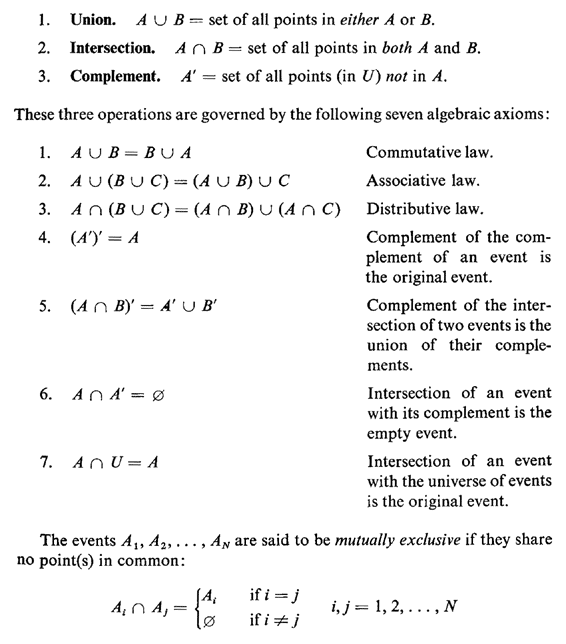Events A1 A2, . . . , AN are said to be collectively exhaustive if they include all points in the universe of events: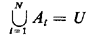A set of events A1, A2,...,AN that are both mutually exclusive and collectively exhaustive contains each point of the sample space U in one and only one of the events Ai

Given these notions, we can more carefully define a sample space as follows:

Definition: A sample space is the finest-grained, mutually exclusive, collectively exhaustive listing of all possible outcomes of an experiment.

The first (and perhaps most important) step in constructing a probabilistic model consists of identifying the sample space for the corresponding experiment.

Example 1: Stick Cutting

A simple example drawn from geometrical probability will serve to illustrate some of these ideas. Suppose that two points are marked (in some nondeterministic way) on a stick of length I meter.

a. Define the sample space for this experiment.

b. Identify the event, "The second point is to the left of the first point."

C. Suppose that the stick is cut at the marked points. Identify the event, "A triangle can be formed with the resulting three pieces."

Solution:

a. Call the first point x1 and the second x2. Since we are given no information about x1 and x2 other than that each is between 0 and 1, the sample space is the collection of points in the unit square shown in Figure 2.1.

b. The event indicated, call it E1, corresponds to (x1 > x2). This set of points lies in the triangular region of the sample space below the line x2 = x1.

c. Let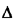be the event that a triangle can be formed. Identification of A in the (x1, x2) sample space requires a bit more care than in the case of the event E1 = (x1 > x2). Suppose that, in fact, x1 > x2. Then the three stick lengths are x2, x1 - x2, and 1 - x1. For a triangle to be formed, each length must be less than 1/2: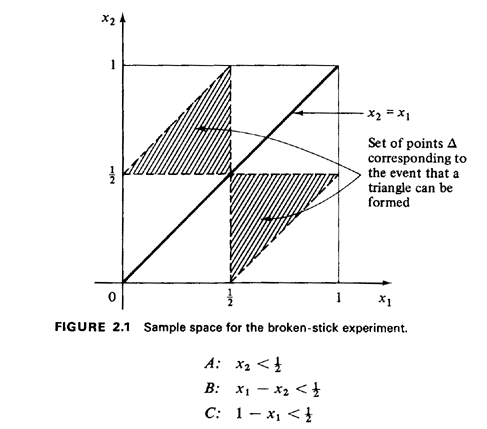So, given x1 > x2,is composed of the set of points that simultaneously satisfies these three inequalities or, equivalently, the set of points in

The resulting set is contained in the lower triangle of area 1/8 in the sample space. Now suppose that (X1X2)=E'1 Here we can invoke symmetry. A current dictionary defines symmetry as "similarity of form or arrangement on either side of a dividing line or plane; correspondence of opposite parts in size, shape, and position."1 Since the labeling of points x1 and x2 was totally arbitrary, there exists symmetry about the line x1 = x2. Thus, we obtain a similar triangle of area 1/8 above the line x1 = x2.

As one final point, we may wish to express A in terms of the algebra of events. If we define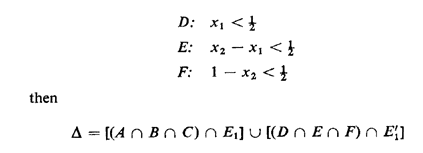In modeling experiments, extreme care must be given to a precise interpretation of the word statement. Statements that may at first sound the same may actually imply markedly different experiments; or, statements may simply be imprecise and ambiguous. A famous illustration of this in a geometrical setting, known as Bertrand's paradox (1907), yields three different answers to the question: What is the probability that a "random chord" of a circle of unit radius has a length greater than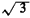the side of an inscribed equilateral triangle ?2 Each of the three solutions, which we will develop in Chapter 3, is "correct" since each involves a different interpretation of that difficult word "random."

To strengthen your understanding of word statements as they relate to concepts of sample space and event, try the following two exercises:

Exercise 2.1: Stick Breaking, mod 2 Repeat parts (a) and (c) of the triangle problem described in Example 1, given that the problem statement is changed as follows: "A point is marked (in some random way) on a stick of length 1 meter. Then a second point is marked on the stick (in some random way) to the left of the first point."

Exercise 2.2: Stick Breaking, Still Again! Repeat parts (a), (b), and (c) of the triangle problem given that the problem statement is changed as follows: "A point is marked (in some random way) on a stick of length 1 meter. The stick is then cut at that point. Another point is marked (in some random way) on the larger of the two resulting stick pieces."

1 Webster's New World Dictionary of the English Language, Second College Edition, World Publishing Co., New York, 1974, p. 1442.

2 J. Bertrand, Calcul des probabilities, Paris, 1907